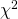## Neutrino masses

In our last global fit, we computed bounds on the absolute scale of neutrino masses using data from the decay experiment KATRIN, from neutrinoless double decay probes (KamLAND-ZEN, GERDA and CUORE) and from cosmology. The results are shown here (see this paper for an explanation of the first figure):Read More →

## 2018

Here you find a summary of the 2018 results. We were obtaining a preference of for normal ordering. Download all the figures here (tar.gz) or here (zip). Our 2018 global fit of neutrino oscillation parameters is published here: @article{deSalas:2017kay, author = “de Salas, P. F. and Forero, D. V. and Ternes, C. A. and T{\’o}rtola, M. and Valle, J. W. F.”, title = “{Status of neutrino oscillations 2018: 3 hint for normal mass ordering and improved CP sensitivity}”, journal = “Phys. Lett.”, volume = “B782”, year = “2018”, pages = “633-640”, doi = “10.1016/j.physletb.2018.06.019”, eprint = “1708.01186”, archivePrefix = “arXiv”, primaryClass = “hep-ph”, SLACcitation =Read More →

##profiles

In the following table you can find the text-readable profiles that you can use to reproduce our 1D and 2D plots. All the files are normalized so that the best fit (in both normal and inverted ordering) always corresponds to . 1D profiles The 1D profiles are written in the format parameter_value chi^2(NO) chi^2(IO) parameter 1D profile dm21_profile.dat dm31_profile.dat sq12_profile.dat sq13_profile.dat sq23_profile.dat del_profile.datRead More →

## The importance of priors

The problem Since 2016, a lot of authors tryed to extract information on the neutrino mass ordering using cosmological data, alone and in combination with neutrino oscillation constraints. One of the most debated topics regards the parameterization and the priors that should be used when describing the neutrino mass sector in such kind of analyses. Simpson et al. used logarithmic priors on the three neutrino masses (, , ) to describe the parameter space and found a strong preference for normal ordering which was never noticed in other analyses (see also Schwetz et al.). Neutrino oscillation data, however, are only sensitive to the squared-mass differences,Read More →

## Neutrino mass ordering

In this page we briefly resume the status of the mass ordering presented here and updated here. The results of the global analysis are here. Masses and ordering The neutrino masses can be ordered in two ways: normal ordering (NO): the lightest neutrino is , the one with the largest mixing with the electron neutrino flavor, and ; inverted ordering (IO): the lightest neutrino is , the one with the smallest mixing with the electron neutrino flavor, and . The mass ordering can be determined measuring the sign of or singling out each of the masses of the three neutrino mass eigenstates. Indirect constraints mayRead More →

## Global fit of oscillation parameters

On this page we present the result of our global fit of neutrino oscillation parameters. The full text can be found in our paper. In the menu on the left hand side you can also obtain information on the experiments analyzed separately. There we also summarize all of the datasets included in our global fit. In the following we present the numerical values of our analysis for each of the parameters. In our global fit of neutrino oscillation data we obtain preference for normal neutrino mass ordering with which corresponds to a preference of . parameter best fit range range : [ eV] 7.50 7.11–7.93Read More →